Next: Numerical simulation Up: Examples Previous: Examples

## Average Group Activation

In order to calculate the average group activation, we model the individual subject activation as being normally distributed according to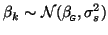where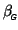represents the average group activation and is usually estimated as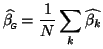and where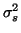denotes the between-subject variance.

We will model the first-level within-subject covariances to be subject-specific and model the between-subject variances (from the group mean) as equal across the group. That is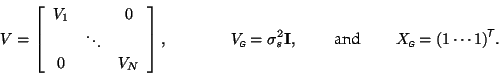Then the adjusted second-level covariance matrix is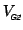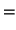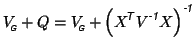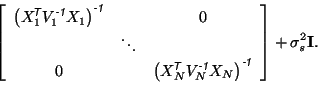Define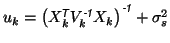, which is the sum of the within- and between-subject covariances. Then the estimate of the group parameter writes as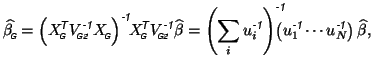(16)

¥ where the inverse sum over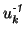s is the associated variance.

Hence we see that in the general framework, the mean group activation parameter is a weighted average of the combined subject- specific activations, where the weights are inversely proportional to the subject-specific variances. This adjustment is advantageous in the case where the individual time-series model does not fit well for a particular subject,, generating an unusual value for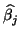(an outlier) but also a large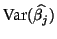. If no correction for the first-level variance is done, then this outlier can significantly affect the estimation of the group (between-subject) variance. If, however, this first-level correction is performed, the increased variance in this parameter will effectively de-weight the contribution of this outlier to the group variance estimate, since we use General Least Squares estimation.

In the much simpler case, where the within-subject covariances are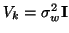, and the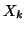are normalised, such that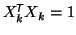for all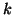, then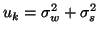and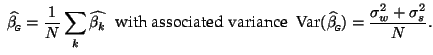(17)

The test for significance is then carried out in a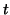-test where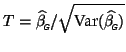has an approximate-distribution.

SubsectionsNext: Numerical simulation Up: Examples Previous: Examples
Christian Beckmann 2003-07-16